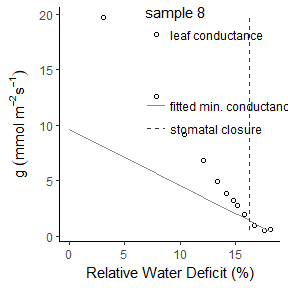# A toolbox for the analysis of pressure volume and leaf drying curves

Experimentally obtained pressure volume and leaf drying curves are widely used for understanding and predicting the strategies of plants to cope with drought stress. By the use of this package, the efficient and reproducible analysis and display of entire experimental datasets is enabled. The methodology of analysing pressure volume and leaf drying curves is well worked out (e.g. Bartlett et al. 2012 and Schuster et al. 2017) and has been adapted by this package.

The package contains two datasets obtained experimentally on Brassica oleracea var. gongylodes for the manufacturing of pressure volume and leaf drying curves and the weather data during the experiments:

pressure_volume_data
leaf_drying_data
weather_data

## Pressure volume curve analysis routine

Pressure volume curves are determined by plotting inversely transformed water potential $$(-1/bar)$$ as a function of relative water deficit ($$RWD$$, $$percentage$$). To determine the turgor loss point by the use of this package, calculate $$RWD$$ first:

pv <- RelativeWaterDeficit(pressure_volume_data)

$$RWD$$ is calculated as:

$$RWD = 100 - 100 * ((FW - DW) (FWs - DW)^{-1})$$

whereas $$FW$$ = fresh weight, $$DW$$ = dry weight and $$FWs$$ = fresh weight at water saturation.

Before continuing, check the raw data for an initial plateau. If the exponential decline does not onset directly, fitting might not succeed.

#### Turgor Loss Point

The function TurgorLossPoint fits the data using the Gauss-Newton algorithm of nls() to a combined exponential and linear model. The exponential and linear parts are extracted and RWD at turgor loss point is localized at their point of minimum distance. The result is outputted as a list and a plot:

pv <- RelativeWaterDeficit(pressure_volume_data)
TurgorLossPoint(pv)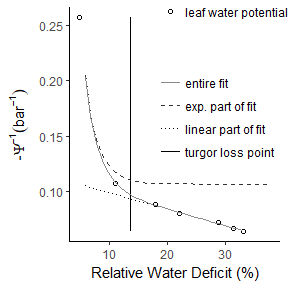#### Osmotic Potential

The function OsmoticPot allows the calculation of water potential at turgor loss point, osmotic potential at full saturation and apoplastic fraction.

First, RWD at turgor loss point is derived by the function TurgorLossPoint. The osmotic potential is then derived by fitting a linear regression line with the Gauss-Newton algorithm of nls() to the water potential data following the turgor loss point. The y- and x-axis intercept of the regression line gives the osmotic potential at full hydration (op.full.sat) and the apoplastic fraction (apo.fract), respectively. Water potential at turgor loss point equals the value of the osmotic potential fit at the relative water deficit at turgor loss point:

pv <- RelativeWaterDeficit(pressure_volume_data)
OsmoticPot(pv)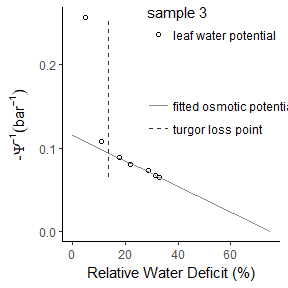#### Modulus of Elasticity

Modulus of elasticity can be determined by the function ModElasticity. Relative water deficit at turgor loss point and osmotic potential are first calculated via the functions ‘TurgorLossPoint’ and ‘OsmoticPot’.

Pressure potential is derived by subtracting osmotic potential from water potential. The part of the pressure potential prior the turgor loss point is then fitted linearly and the modulus of elasticity (M.Elasticity) equals the slope of the fitted line:

ModElasticity(pv)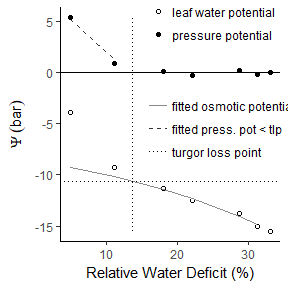## Leaf drying curve analysis routine

Leaf drying curves show leaf conductance as a function of leaf relative water content. Leaf conductance initially drops exponentially and settles to linear water loss after stomata close. To obtain a leaf drying curve, water loss per time unit and leaf area needs to be transformed to conductance by the use of the driving force for transpirational water loss, e.g. the vapor pressure deficit.

#### Driving force for transpiration

Vapor pressure deficit is calculated by the humidity and temperature during the experiment which need to be allocated to the weight loss measurements fist. The function WeatherAllocation calculates averages within a measurement period by approximate integration and normalization of the weather as a function of time:

library(pvldcurve)
data <- WeatherAllocation(leaf_drying_data, weather_data)

Mole fraction vapor pressure deficit can be calculated individually by the use of the function VaporPressureDeficit as:

data <- VaporPressureDeficit(data)

$$VPD =(1 - RH / 100) * (VPsat / AP)$$

whereas $$RH$$ = relative humidity $$(percentage)$$, $$AP$$ = atmospheric pressure $$(kPA)$$, $$VPsat$$ = saturation vapor pressure $$(kPA)$$, whereas:

$$VPsat = 0.61121 * exp ((18.678 - T * 234.5^{-1}) (T * 257.14 + T))$$

or:

data <- SaturationVaporPressure(data)

where $$T$$ = air temperature $$(degree Celsius)$$. Atmospheric pressure at the experimental site can be specified as an argument. It is by default 101.325 $$kPA$$.

#### Transpiration

Transpiration $$(mmol s^{-1} m^{-2})$$ is calculated by the function Transpiration as:

data <- Transpiration(data,
fresh.weight = "fresh.weight")

$$T = \Delta FM * 1000 (\Delta t * 60 * LA * 2 /10000 * 18.01528)^{-1}$$

whereas $$T$$ = transpiration, $$\Delta FM$$ = reduction of fresh weight $$(g)$$, $$\Delta t$$ = time unit $$(min)$$, $$LA$$ = single-sided leaf area $$(cm^2)$$ $$\Delta FM$$ can be calculated by WeightDifference and $$\Delta t$$ by TimeInterval:

data:wd <- WeightDifference(data)
data_ti <- TimeInterval(data)

#### Conductance

The function Conductance calculates mole-based conductance $$(mmol s^{-1} m^{-2})$$ as:

$$g = Tp / VPD$$

It doesn’t require the prior transformation of data but works with the raw data :

data <- Conductance(data,
fresh.weight = "fresh.weight")

Concentration based conductance $$(mm s^{-1})$$ is derived from mole-based conductance as:

$$g(conc) = g * R * (T + 273.15) / AP / 1000$$

where $$R$$ = gas constant.

data <- Conductance(data,
fresh.weight = "fresh.weight",
driving.force = "conc")

#### Stomatal Closure

The point of stomatal closure is reached when the leaf drying curve settles irreversibly to linear water loss. First, calculate RWD based on the interval between two measurements and time since start $$(min)$$ of the experiment:

data <- RWDInterval(leaf_drying_data,
fresh.weight = "fresh.weight")
data <- TimeSinceStart(data)

To find the point of stomatal closure, the conductances by time since start curves are fitted using the Gauss-Newton algorithm of nls() to a combined exponential and linear model. The exponential and linear parts are extracted and time since start at stomatal closure is localized at the point where the slope of the exponential part of the fit is higher than a threshold value.

The threshold value is calculated by the use of the parameter b of the exponential part of the fit $$(a * exp(b * x))$$: $$-(b^2 * sens)$$. The sensitivity argument ($$sens$$) is 60 by default and can be specified individually using the argument ‘threshold’.

RWD at stomatal closure is then calculated by linear regression of RWD and time since start.

Before using this function, check the raw data for an initial plateau. If the exponential decline does not onset directly, fitting might not succeed. In the inherent dataset, plateauing data points are marked in a separate column.

data <- WeatherAllocation(leaf_drying_data, weather_data)
data <- TimeSinceStart(data)
data <- RWDInterval(data,
fresh.weight = "fresh.weight")
data <- data[data$fw.plateau != "yes",] data <- Conductance(data, fresh.weight = "fresh.weight") StomatalClosure(data)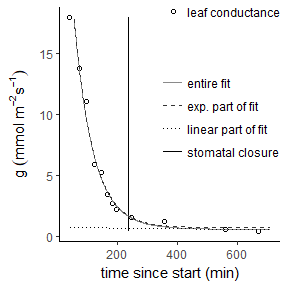Removing noises in the fresh weight values is recommend prior using StomatalClosure(). The function FittedFW will do so via fitting of the fresh weight values to a combined exponential and linear model using the port algorithm of nls(): data <- WeatherAllocation(leaf_drying_data, weather_data) data <- TimeSinceStart(data) data <- data[data$fw.plateau != "yes",]
data <- FittedFW(data)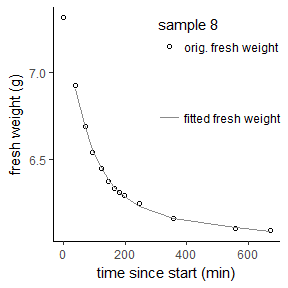Thereby, calculation of stomatal closure is improved:

data <- WeatherAllocation(leaf_drying_data, weather_data)
data <- TimeSinceStart(data)
data <- data[data$fw.plateau != "yes",] data <- FittedFW(data, graph = FALSE) data <- RWDInterval(data, fresh.weight = "fitted.fw") data <- Conductance(data, fresh.weight = "fitted.fw") StomatalClosure(data)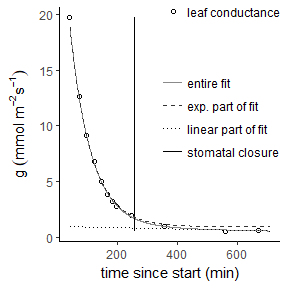#### Minimum conductance Minimum conductance is calculated with the function Gmin by the leaf drying curve in three ways. First, The coordinates of stomatal closure are determined via the function StomatalClosure(). Then, conductance data including and following stomatal closure is extracted and the average is taken (mean.gmin). A linear regression is applied to the data including gmin at stomatal closure, which is determined by the function StomatalClosure(), and the y axis intercept (gmin.full.sat) and the coordinate at the RWD point of stomatal closure (lin.gmin) are calculated from the function. data <- WeatherAllocation(leaf_drying_data, weather_data) data <- TimeSinceStart(data) data <- data[data$fw.plateau != "yes",]
data <- FittedFW(data, graph = FALSE)
data <- RWDInterval(data, fresh.weight = "fitted.fw")
data <- Conductance(data, fresh.weight = "fitted.fw")

Gmin(data)